## 6.1  Perturbation Theory with Lie Series

Given a system, we look for a decomposition of the Hamiltonian in the form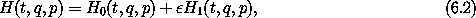where H0 is solvable. We assume that the Hamiltonian has no explicit time dependence; this can be ensured by going to the extended phase space if necessary. We also assume that a canonical transformation has been made so that H0 depends solely on the momenta: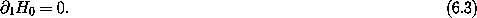We carry out a Lie transformation and find the conditions that the Lie generator W must satisfy to eliminate the order-terms from the Hamiltonian.

The Lie transform and associated Lie series specify a canonical transformation: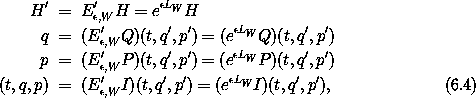where Q = I1 and P = I2 are the coordinate and momentum selectors and I is the identity function. Recall the definitions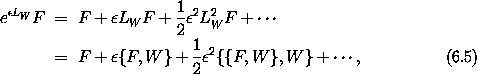with LW F = { F, W }.

Applying the Lie transformation to H gives us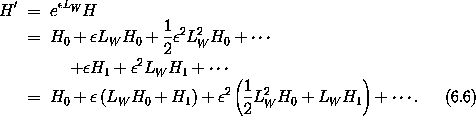The first-order term inis zero if W satisfies the condition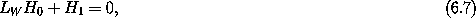which is a linear partial differential equation for W. The transformed Hamiltonian is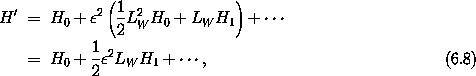where we have used condition (6.7) to simplify the2 contribution.

This basic step of perturbation theory has eliminated terms of a certain order (order) from the Hamiltonian, but in doing so has generated new terms of higher order (here2 and higher).

At this point we can find an approximate solution by truncating Hamiltonian (6.8) to H0, which is solvable. The approximate solution for given initial conditions (t0, q0, p0) is obtained by finding the corresponding (t0, q'0, p'0) using the inverse of transformation (6.4). Then the system is evolved to time t using the solutions of the truncated Hamiltonian H0, giving the state (t, q', p'). The phase-space coordinates of the evolved point are transformed back to the original variables using the transformation (6.4) to state (t, q, p). The approximate solution is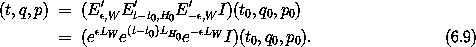If the Lie transform E', W = eLW must be evaluated by summing the series, then we must specify the order to which the sum extends.

Assuming everything goes okay, we can imagine repeating this process to eliminate the order-2 terms and so on, bringing the transformed Hamiltonian as close as we like to H0. Unfortunately, there are complications. We can understand some of these complications and how to deal with them by considering some specific applications.